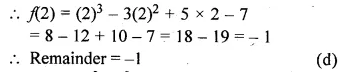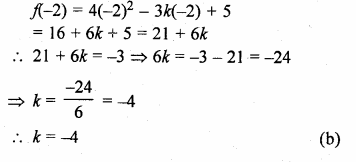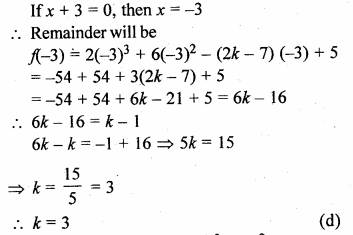## ML Aggarwal Class 10 Solutions for ICSE Maths Chapter 6 Factorization MCQS

These Solutions are part of ML Aggarwal Class 10 Solutions for ICSE Maths. Here we have given ML Aggarwal Class 10 Solutions for ICSE Maths Chapter 6 Factorization MCQS

More Exercises

Choose the correct answer from the given four options (1 to 5) :

Question 1.
When x3 – 3x2 + 5x – 7 is divided by x – 2,then the remainder is
(a) 0
(b) 1
(c) 2
(d) – 1
Solution:
f(x) = x3 – 3x2 + 5x – 7
g(x) = x – 2, if x – 2 = 0, then x = 2
Remainder will beQuestion 2.
When 2x3 – x2 – 3x + 5 is divided by 2x + 1, then the remainder is
(a) 6
(b) – 6
(c) – 3
(d) 0
Solution:
f(x) = 2x3 – x2 – 3x + 5
g(x) = 2x + 1Question 3.
If on dividing 4x2 – 3kx + 5 by x + 2, the remainder is – 3 then the value of k is
(a) 4
(b) – 4
(c) 3
(d) – 3
Solution:
f(x) = 4x2 – 3kx + 5
g(x) = x + 2
Remainder = – 3
Let x + 2 = 0, then x = – 2
Now remainder will beQuestion 4.
If on dividing 2x3 + 6x2 – (2k – 7)x + 5 by x + 3, the remainder is k – 1 then the value of k is
(a) 2
(b) – 2
(c) – 3
(d) 3
Solution:
f(x) = 2x3 + 6x2 – (2k – 7)x + 5
g(x) = x + 3
Remainder = k – 1Question 5.
If x + 1 is a factor of 3x3 + kx2 + 7x + 4, then the value of k is
(a) – 1
(b) 0
(c) 6
(d) 10
Solution:
f(x) = 3x3 + kx2 + 7x + 4
g(x) = x + 1
Remainder = 0Hope given ML Aggarwal Class 10 Solutions for ICSE Maths Chapter 6 Factorization MCQS are helpful to complete your math homework.

If you have any doubts, please comment below. Learn Insta try to provide online math tutoring for you.